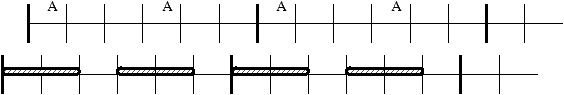Optimal Carrier Sharing in Wireless TDMA

Ed Coffman

New Jersey Institute of Technology

Algorithms Seminar

February 4, 1999

[summary by Philippe Robert]

A properly typeset version of this document is available in postscript and in pdf.

If some fonts do not look right on your screen, this might be fixed by configuring your browser (see the documentation here).

Abstract
An important design issue in implementing Dynamic Channel Assignment in Time Division Multiple Access (TDMA) wireless networks is whether resource allocation should be done on a per-carrier basis or at the time slot level. Resource allocation at the time slot level is seriously hampered by the lack of synchronization between base stations in distinct cells. We present simple greedy packing algorithms which overcome this obstacle by clustering calls. The results suggest that the algorithms are nearly optimal, and that little extra performance can be gained either by allowing the rejection of calls or by repacking.

TDMA (Time Division Multiple Access) systems supply most digital cellular services (GSM for example). The available spectrum is divided into carriers (frequency bands) and each of these is time slotted. A carrier is slotted and up to n calls can be time-multiplexed at one time (for example there are 8 time-slots per carrier for GSM). When n calls are active, call requests are rejected since no queueing is possible.

The calls in a cell A can share any given carrier. A carrier assigned to the cell A will not be assigned to another cell close enough to interfere with its calls. This talk presents algorithms ensuring that a neighboring cell B is allowed to time-share the carrier with cell A. The sharing mechanism must take into account that the slots in cells A and B are not perfectly synchronized. To avoid interference, the slots assigned to cell A are not allowed to overlap slots assigned to cell B.Clearly, to maximize the acceptance rate of call s, time-slot allocation should try to cluster separately A-calls and B-calls. In the above figure the hatched rectangles indicate time slots which cannot be used by cell B. In this case clustering of A-calls would make 3 slots available instead of 2. The time-slots are represented by a circle with n slots.

Definition 1   An algorithm of slot allocation is greedy if a new call is accepted if there is an available slot.

A
repacking algorithm can reallocate the time-slots (i.e., repack) in order to admit a new call.

### Some Examples of Assignment Algorithms

• Clustering algorithms Cn. A call is packed in the first available slot found in a scan of the current state clockwise from slot (1,2) if an A-call and counterclockwise from slot (2n-2, 2n-1) if a B-call. This algorithm tries to separate as much as possible A and B-calls.

• Weighting algorithms Wn. Each slot carries a weight. At arrival, the quantity d0 is added to the A-slot receiving the new call and d1 to neighboring slots, d2 to the slots at distance 2,.... The quantity d1' is added to the B-slots covered by the slot of the new call, d2' to the B-slots at distance 2, etc. An analogous procedure applies to the B-call arrivals. Weight changes are reversed at departure times.

• Repacking algorithm Rn. Pack A and B calls in separate clusters whenever necessary.
A stochastic model is considered, the calls in cells A and B arrive according to a Poisson process with parameter lA and lB respectively. Holding times are independent with rate µ in each cell. For X=A, B, rX=lX/µ and pX denotes the probability of rejecting an X-call. The overall probability to be minimized is then
pArA+pBrB
rA+rB
.
The classical method to find the optimal algorithm to minimize the blocking probability is to solve the associated Bellman equation. As usual this equation is very hard to solve explicitly except when the state space is quite small.

Some numerical values are presented and discussed in the case n=8. The main mathematical result is the following theorem.
Theorem 1   If all optimal algorithms are greedy and if holding times are i.i.d. exponentials, then the algorithm Wn is optimal for 1£ n£ 6.
The proof relies on a coupling argument.

## References


Borst (S. C.), Coffman (E. G.), Gilbert (E. N.), Whiting (P. A.), and Winkler (P. M.). -- Optimal carrier sharing in wireless TDMA, September 1999.

This document was translated from LATEX by HEVEA.